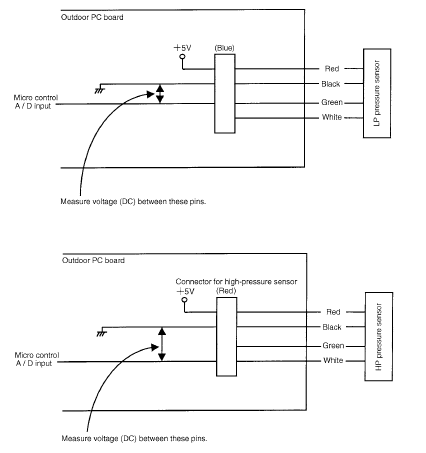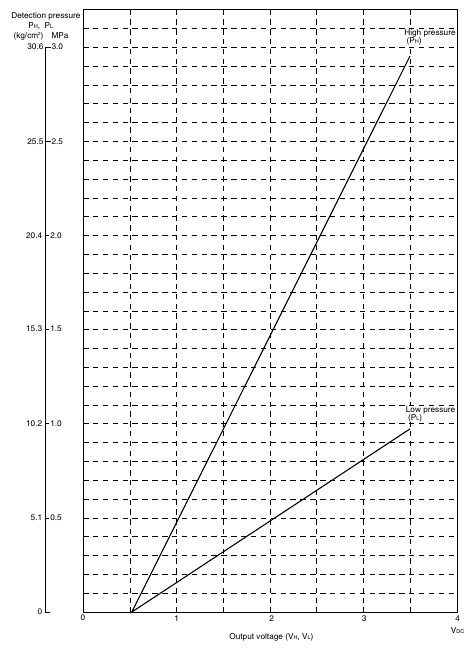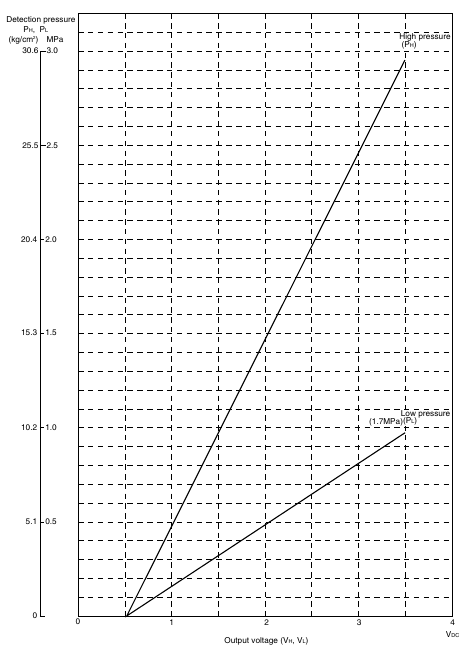Daikin VRV – Pressure Sensor

Voltage Measuring PointWhen the color of harness is not as mentioned above, find the measuring point by following procedure.
1. Black is GND.
2. Find DC5V line.
3. The remained wire is the sensing section.
(For the same pressure, higher output voltage can be detected with low-pressure sensor, while lower output voltage detected with high pressure sensor.)

Voltage Characteristic of Pressure Sensor (R22, R407C)
High pressure PH = (VH – 0.5) × 0.98
Low pressure P L = (VL – 0.5) × 0.98 / 3
PH : Detection pressure [High pressure side] MPa
PL : Detection pressure [Low pressure side] MPa
VH : Output voltage [High pressure side] V DC
VL : Output voltage [Low pressure side] VDCVoltage Characteristic of Pressure Sensor (R410A)
PH = 1.38V ? 0.69
PL = 0.57V ? 0.28
PH : Detection pressure [High pressure side] MPa
PL : Detection pressure [Low pressure side] MPa
VH : Output voltage [High pressure side] V DC
VL : Output voltage [Low pressure side] VDC